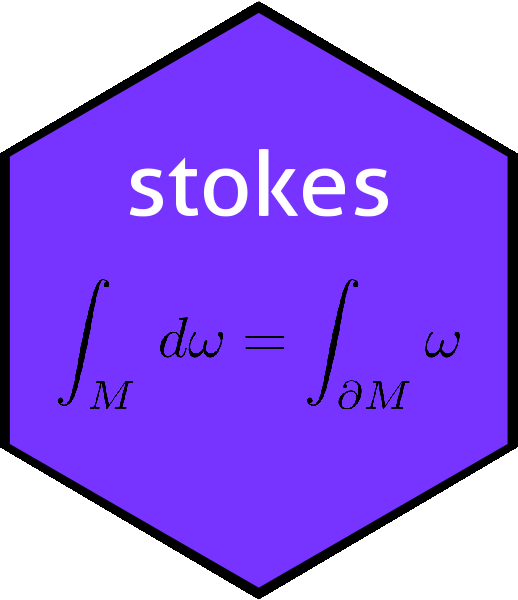# Objects dx, dy, and dz in the stokes packagedx <- d(1)
dy <- d(2)
dz <- d(3)

Convenience objects dx, dy, and dz are discussed here. The conjugate basis vectors i, j and k are discussed in ijk.Rmd.

Spivak, in a memorable passage, states (p89):

Fields and forms

If $$f\colon\mathbb{R}^n\longrightarrow\mathbb{R}$$ is differentiable, then $$Df(p)\in\Lambda^1(\mathbb{R}^n)$$. By a minor modification we therefore obtain a $$1$$-form $$df$$, defined by

$df(p)(v_p)=Df(p)(v).$

Let us consider in particular the $$1$$-forms $$d\pi^i$$ 1. It is customary to let $$x^i$$ denote the function $$\pi^i$$ (on $$\mathbb{R}^3$$ we often denote $$x^1$$, $$x^2$$, and $$x^3$$ by $$x$$, $$y$$, and $$z$$) $$\ldots$$ Since $$dx^i(p)(v_p)=d\pi^i(p)(v_p)=D\pi^i(p)(v)=v^i$$, we see that $$dx^1(p),\ldots,dx^n(p)$$ is just the dual basis to $$(e_1)_p,\ldots, (e_n)_p$$.

- Michael Spivak, 1969 (Calculus on Manifolds, Perseus books). Page 89

Spivak goes on to observe that every $$k$$-form $$\omega$$ can be written $$\omega=\sum_{i_1 < \cdots < i_k}\omega_{i_1,\ldots i_k}dx^{i_1}\wedge\cdots\wedge dx^{i_k}$$. If working in $$\mathbb{R}^3$$, we have three elementary forms $$dx$$, $$dy$$, and $$dz$$; in the package we have the pre-defined objects dx, dy, and dz. These are convenient for reproducing textbook results. We start with some illustrations of the package print method.

dx
## An alternating linear map from V^1 to R with V=R^1:
##        val
##  1  =    1

This is somewhat opaque and difficult to understand. It is easier to start with a more complicated example: take $$dx\wedge dy -7dx\wedge dz + 3dy\wedge dz$$:

dx^dy -7*dx^dz + 3*dy^dz
## An alternating linear map from V^2 to R with V=R^3:
##          val
##  1 3  =   -7
##  2 3  =    3
##  1 2  =    1

We see three rows for the three elementary components. Taking the row with coefficient $$-7$$ [which would be $$-7dx\wedge dz$$], this maps $$\left(\mathbb{R}^3\right)^2$$ to $$\mathbb{R}$$ and we have

$(-7dx\wedge dz)\left(\begin{pmatrix} u_1\\u_2\\u_3\end{pmatrix}, \begin{pmatrix}v_1\\v_2\\v_3\end{pmatrix}\right)= -7\det\begin{pmatrix}u_1&v_1\\u_3&v_3\end{pmatrix}$

Armed with this familiar fact, we can interpret $$dx$$ as a map from $$\left(\mathbb{R}^3\right)^1$$ to $$\mathbb{R}$$ with

$dx\left(\begin{pmatrix} u_1\\u_2\\u_3\end{pmatrix} \right)= \det\begin{pmatrix}u_1\end{pmatrix}=u_1$

or, in other words, $$dx$$ picks out the first component of its vector (as the print method gives, albeit obscurely). This is easily shown in the package:

as.function(dx)(c(113,3,6))
##  113

We might want to verify that $$dx\wedge dy=-dy\wedge dx$$:

dx ^ dy == -dy ^ dx
##  TRUE

# Elementary forms and the print method

The print method is configurable and can display kforms in symbolic form. For working with dx dy dz we may set option kform_symbolic_print to dx:

options(kform_symbolic_print = 'dx')

Then the results of calculations are more natural:

dx
## An alternating linear map from V^1 to R with V=R^1:
##  + dx
dx^dy + 56*dy^dz
## An alternating linear map from V^2 to R with V=R^3:
##  + dx^dy +56 dy^dz

However, this setting can be confusing if we work with $$dx^i,i>3$$, for the print method runs out of alphabet:

rform()
## An alternating linear map from V^3 to R with V=R^7:
##  +6 dy^dNA^dNA +5 dy^dNA^dNA -9 dNA^dNA^dNA +4 dx^dz^dNA +7 dx^dNA^dNA -3 dy^dz^dNA -8 dx^dNA^dNA +2 dx^dy^dNA + dx^dNA^dNA

Above, we see the use of NA because there is no defined symbol.

## The Hodge dual

Function hodge() returns the Hodge dual:

hodge(dx^dy + 13*dy^dz)
## An alternating linear map from V^1 to R with V=R^3:
##  +13 dx + dz

Note that calling hodge(dx) can be confusing:

hodge(dx)
##  1

This returns a scalar because dx is interpreted as a one-form on one-dimensional space, which is a scalar form. One usually wants the result in three dimensions:

hodge(dx,3)
## An alternating linear map from V^2 to R with V=R^3:
##  + dy^dz

This is further discussed in the dovs vignette.

## Other ways to create the elementary one-forms

It is possible to create these objects using package idiom:

d(1) == dx
##  TRUE

## Basis vectors

### Package dataset

Following lines create dx.rda, residing in the data/ directory of the package.

save(dx,dy,dz,file="dx.rda")

1. Spivak introduces the $$\pi^i$$ notation on page 11: “if $$\pi\colon\mathbb{R}^n\longrightarrow\mathbb{R}^n$$ is the identity function, $$\pi(x)=x$$, then [its components are] $$\pi^i(x)=x^i$$; the function $$\pi^i$$ is called the $$i^\mathrm{th}$$ projection function↩︎